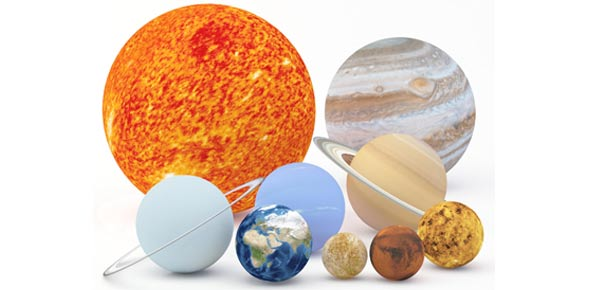# Chapter 25 & Chapter 26 Astronomy Test

33 Questions | Total Attempts: 145SettingsI hate this class. . .

• 1.
A billion solar mass black hole has a radius of only _____ (in A.U)
• A.

• B.

• C.

• 2.
What determines whether colliding gallaxies will stick and merge or simply pass through eachother?
• A.

Time

• B.

Crowding

• C.

Space

• 3.
The Black hole at the center of active galaxie or quasar requires ______ to radiate massive amounts of energy
• A.

Matter

• B.

Energy

• C.

Fuel

• 4.
The central regions of active galaxies are fed by ____
• A.

Fuel

• B.

Energy

• C.

Matter

• 5.
A galaxy is at a distance of one billion light years. What is true about the way we see that galaxy?
• A.

We see it 5 billion years away

• B.

We see it one billion years away

• C.

We see it ten billion years away

• 6.
Dark matter is ____ %  of the matter in the universe
• 7.
The best method of determining the mass of nearby spiral galaxies is by:
• A.

Determining its distance

• B.

Determining its rotation curves

• C.

Determining its amount of matter

• 8.
What is the effect on individual stars when galaxies collide?
• A.

No effect

• B.

The combined

• 9.
Collisions between galaxies result in
• A.

Rearranging the stellar contents

• B.

Stars merging

• C.

Rearranging the interstellar conents

• 10.
The most massive galaxies have _____ of times more stars than the milky way.
• 11.
Which type of galaxy is rare in the center of clusters?
• A.

Spiral

• B.

Binary

• C.

Ordinary

• 12.
Why are super-massive galaxies often found at the cores of rich galaxy clusters?
• A.

Cannibalism

• B.

Due to the amount of energy

• C.

Because of gravity

• 13.
In their most energetic phase, a quasar can probably only last
• A.

Two years

• B.

Two million years

• C.

Two billion years

• 14.
________ Galaxies may bridge the gap between spiral galaxies and quasars.
• A.

Elliptical

• B.

Binary

• C.

Seyfret

• 15.
We now believe the majority of mass for most galaxies lies in their dark halos
• A.

True

• B.

False

• 16.
While yet unseen, some gravitational evidence suggests the existence of dark gallaxies
• A.

True

• B.

False

• 17.
Collisions between galaxies have a larger effect on the individual stars
• A.

True

• B.

False

• 18.
Collisions between galaxies are rare, just as in case with stars.
• A.

True

• B.

False

• 19.
Quasars end in a massive explosion as a gamma ray burst.
• A.

True

• B.

False

• 20.
The last quasar probably died out a billion years ago, for none lie within a billion light years of us
• A.

True

• B.

False

• 21.
What does the hubble law imply about the history of the universe?
• A.

It is untrue

• B.

It has always been expanding

• 22.
Recent work with Type I supernovae at great distances suggests the universe may in face be accelerating its expansion, a discovery attributed the newly found ______
• A.

Date

• B.

Age

• C.

Time

• 23.
According to their H-R diagrams turn off points, how old are the oldest globular clusters?
• A.

3-4 billion years

• B.

4-5 billion years

• C.

5-6 billion years

• 24.
In our best current theory, how long ago did the first quasars form?
• A.

11 billion years ago

• B.

13 billion years ago

• C.

15 billion years ago

• 25.
About how many galaxies are estimated to be in the observable universe?
• A.

30 billion

• B.

35 billion

• C.

40 billion

Related TopicsBack to top